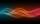Unknown x

If we add to unknown number 21, then divide by 6 and then subtract 51, we get back an unknown number.

What is this unknown number?

Result

x =  -57

Solution:Leave us a comment of example and its solution (i.e. if it is still somewhat unclear...):Be the first to comment!To solve this example are needed these knowledge from mathematics:

Do you have a linear equation or system of equations and looking for its solution? Or do you have quadratic equation?

Next similar examples:

1. One-thirdA one-third of unknown number is equal to five times as great as the difference of the same unknown number and number 28. Determine the unknown number.
2. Unknown numberIdentify unknown number which 1/5 is 40 greater than one tenth of that number.
3. Unknown number 11That number increased by three equals three times itself?
4. Find xSolve: if 2(x-1)=14, then x= (solve an equation with one unknown)
5. Mysterious numberThe magician thinks the number: "The mysterious number is first divided by minus five, dividing the result by three, multiplying the number by ten, and dividing the resulting number by minus four. This gives result 5. Can you reveal the mysterious number?
6. NumberCalculate the integer number which, divided by 34 gives 10 and the rest 25.
7. Dropped sheetsThree consecutive sheets dropped from the book. The sum of the numbers on the pages of the dropped sheets is 273. What number has the last page of the dropped sheets?
8. 15 numberWhat number is smaller (greater) by 15 than its half?
9. EquationSolve the equation: 1/2-2/8 = 1/10; Write the result as a decimal number.
10. What isWhat is the value of the smaller of a pair of numbers for which their sum is 78 and their division quotients are 0.3?
11. Negative in equation2x + 3 + 7x = – 24, what is the value of x?
12. Simple equationSolve for x: 3(x + 2) = x - 18
13. Forest nurseryIn the forest nursery after winter, they found that 1/10 stems died out of them. For them, they land 193 new spruces. How many spruces are in the forest nursery?
14. NormThree workers planted 3555 seedlings of tomatoes in one dey. First worked at the standard norm, the second planted 120 seedlings more and the third 135 seedlings more than the first worker. How many seedlings were standard norm?
15. Equation 29Solve next equation: 2 ( 2x + 3 ) = 8 ( 1 - x) -5 ( x -2 )
16. CakesGrandmother baked cakes. Half of its was poppy, quarter with plum jam and 16 cheesecakes. How many cakes she baked in total?
17. EquatiomSolve equation with negatives: X/(-5) + 2 = -9